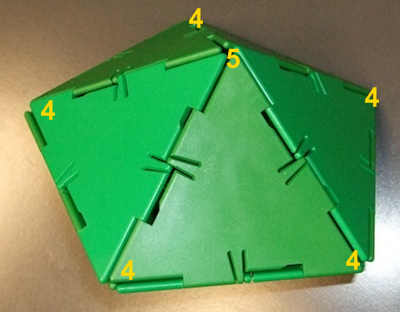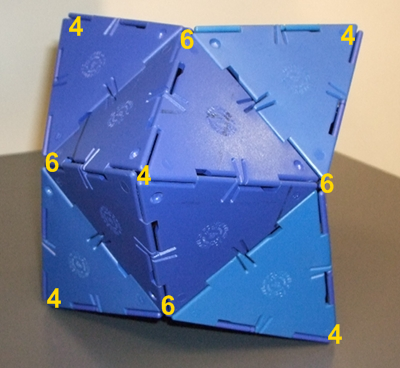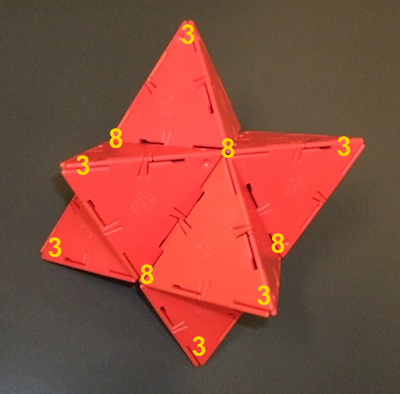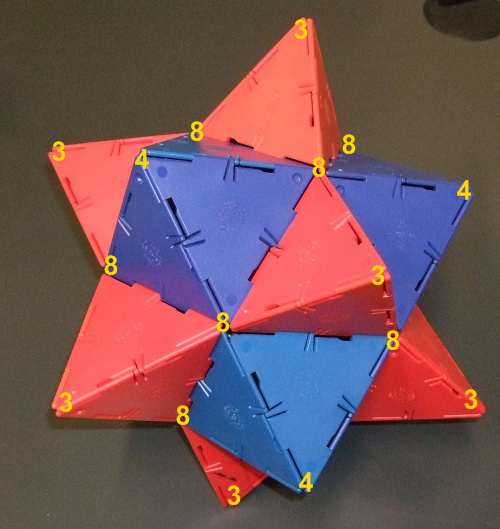# Investigating Solids with Face-transitivity

##### Age 14 to 18

Published 2009

Here are the vertices of the $V_{3,6,6}$ solid labelled to show the types of vertex on each face.
Notice how every triangular face has one $V_3$ vertex and two $V_6$ vertices.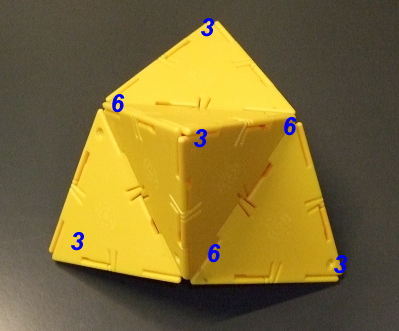Below are the other images from the article with their vertices labelled to show how many faces are meeting at each vertex.